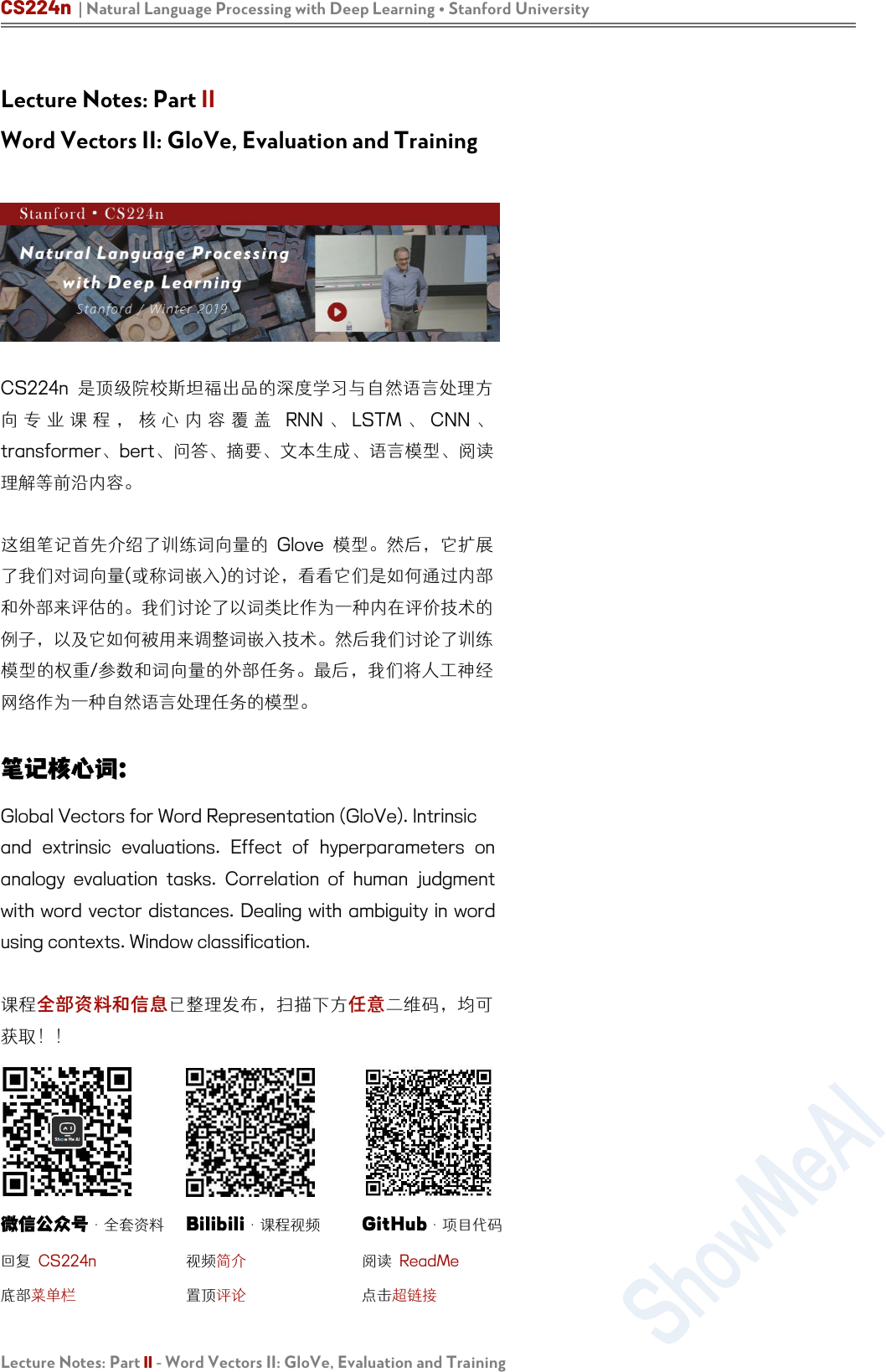CS224n | Natural Language Processing with Deep Learning Stanford University
Lecture Notes: Part II - Word Vectors II: GloVe, Evaluation and Training
Lecture Notes: Part II
Word Vectors II: GloVe, Evaluation and Training
CS224n
RNN LSTM CNN
transformerbert问答要、成、模型

Global Vectors for Word Representation (GloVe). Intrinsic
and extrinsic evaluations. Effect of hyperparameters on
analogy evaluation tasks. Correlation of human judgment
with word vector distances. Dealing with ambiguity in word
using contexts. Window classification.

·全套资料

Bilibili
·课程视频

GitHub
·项目代码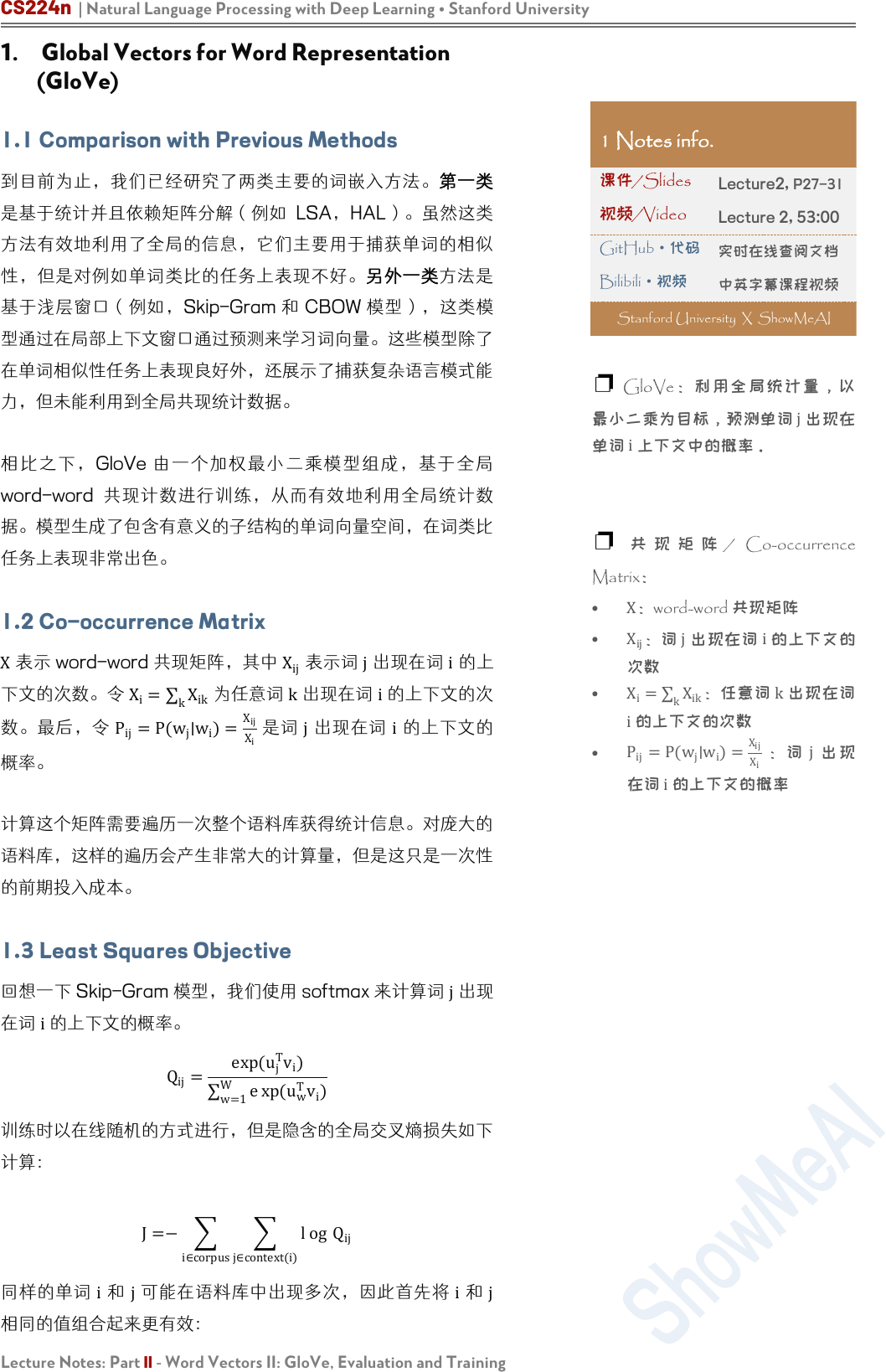CS224n | Natural Language Processing with Deep Learning Stanford University
Lecture Notes: Part II - Word Vectors II: GloVe, Evaluation and Training
1. Global Vectors for Word Representation
(GloVe)
1.1 Comparison with Previous Methods

Skip-Gram CBOW

GloVe
word-word

1.2 Co-occurrence Matrix
X

X
ij

X
i
=
k
X
ik

k

i

P
ij
=P(w
j
∣w
i
)=
X
ij
X
i
j i

1.3 Least Squares Objective

Q
ij
=
exp(u
j
T
v
i
)
w=1
W
e xp(
u
w
T
v
i
)

J=
i∈corpus
j∈context(i)
l og Q
ij
i j i j

1 Notes info.

Lecture2, P27-31

Lecture 2, 53:00
GitHub
·代码

Bilibili·视频

Stanford University X ShowMeAI
GloVe

j

i

/ Co-occurrence
Matrix
X
word-word 共现矩阵
X
ij
：词
j

i

X
i
=
k
X
ik
：任
k

i

P
ij
=P(w
j
∣w
i
)=
X
ij
X
i
j

i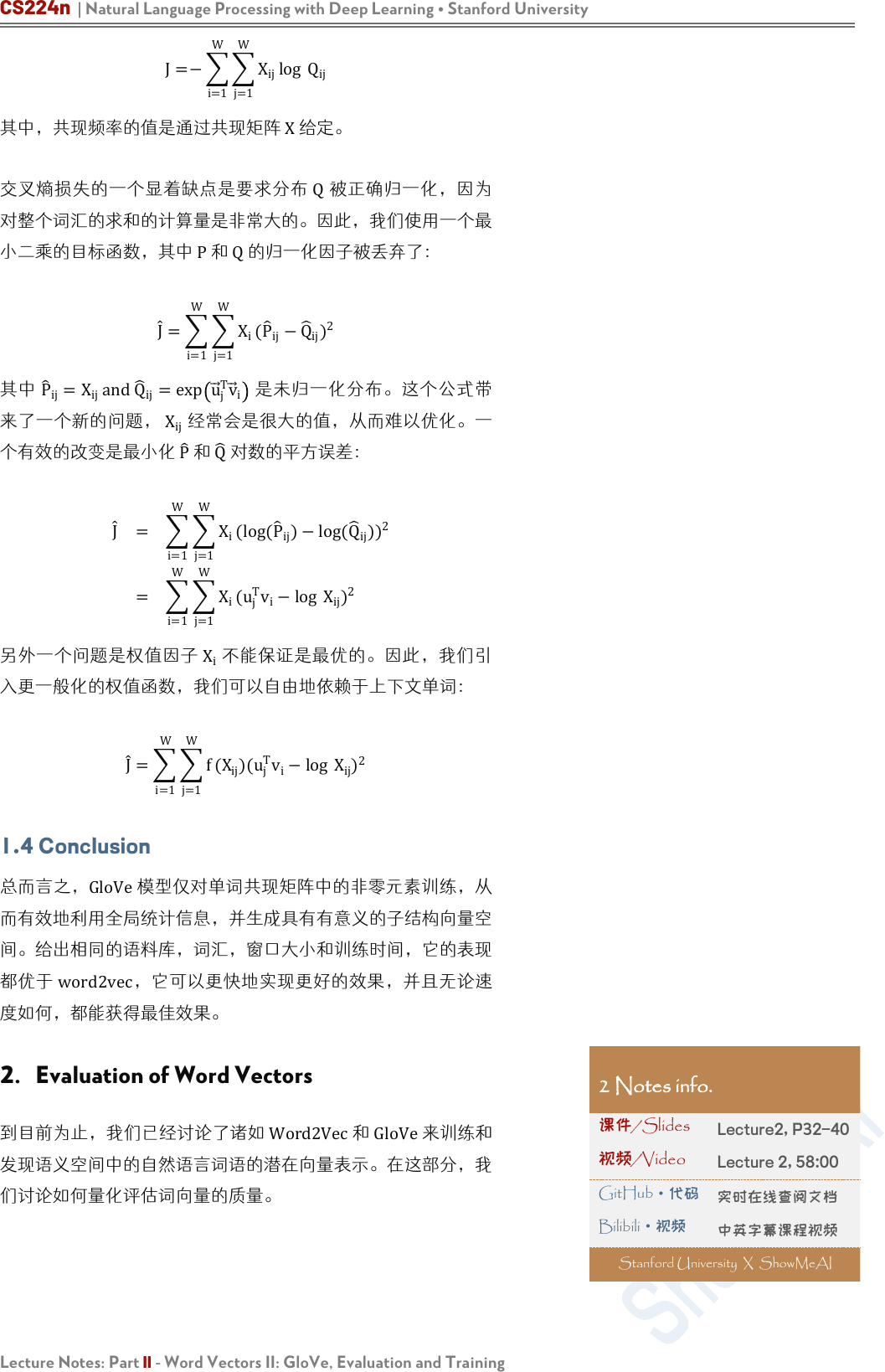CS224n | Natural Language Processing with Deep Learning Stanford University
Lecture Notes: Part II - Word Vectors II: GloVe, Evaluation and Training
J=
i=1
W
j=1
W
X
ij
log Q
ij

Q

J
=
i=1
W
j=1
W
X
i
(
P
ij
Q
ij
)
2
P
ij
=X
ij
andQ
ij
=exp u
j
T
v
i

X
ij

P
Q

J
=
i=1
W
j=1
W
X
i
(log(
P
ij
)log(
Q
ij
)
)
2
=
i=1
W
j=1
W
X
i
(u
j
T
v
i
log
X
ij
)
2
X
i

J
=
i=1
W
j=1
W
f (X
ij
)(u
j
T
v
i
log X
ij
)
2
1.4 Conclusion

2. Evaluation of Word Vectors

Lecture2, P32-40

Lecture 2, 58:00
GitHub
·代码

Bilibili·视频CS224n | Natural Language Processing with Deep Learning Stanford University
Lecture Notes: Part II - Word Vectors II: GloVe, Evaluation and Training
2.1 Intrinsic Evaluation

1. 输入词语
2. 将输入词语转换为词向量
3. 对一个复杂的机器学习系统，使用词向量作为输入
4. 将输出的词向量通过系统映射到自然语言词语上。
5. 生成词语作为答案

3

2.2 Extrinsic Evaluation

Figure 1: The left subsystem
(red) being expensive to train is
modified by substituting with a
simpler subsystem (green) for
intrinsic evaluation. 1CS224n | Natural Language Processing with Deep Learning Stanford University
Lecture Notes: Part II - Word Vectors II: GloVe, Evaluation and Training
2.3 Intrinsic Evaluation Example: Word Vector
Analogies

a:b::c:?

d=argmax
i
(x
b
x
a
+x
c
)
T
x
i
|x
b
x
a
+
x
c
|
x
b
x
a
=
x
d
x
c
queen-king=actress-actor

b
x
a
+
x
c
=x
d
。因确定两个

d


1:


1:
:
2:


2

10 Phoenix Arizona



1:

1:
:

2:

2

1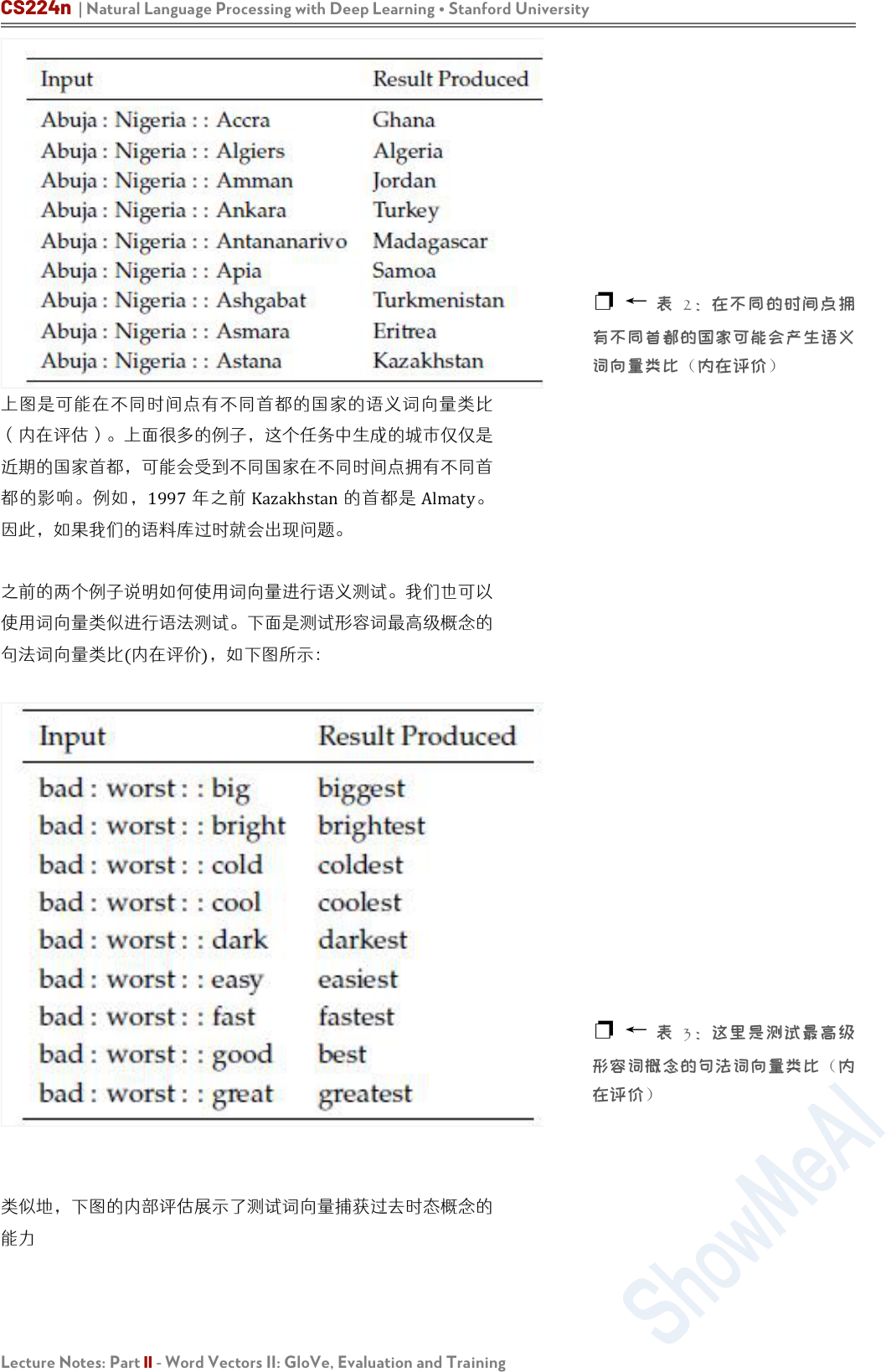CS224n | Natural Language Processing with Deep Learning Stanford University
Lecture Notes: Part II - Word Vectors II: GloVe, Evaluation and Training
（内在评估）。上面很多的例子，这个任务中生成的城市仅仅是

1997 Kazakhstan Almaty

2

3CS224n | Natural Language Processing with Deep Learning Stanford University
Lecture Notes: Part II - Word Vectors II: GloVe, Evaluation and Training
2.4 Intrinsic Evaluation Tuning Example:
Analogy Evaluations

4
GloVe 使
8

2

5CS224n | Natural Language Processing with Deep Learning Stanford University
Lecture Notes: Part II - Word Vectors II: GloVe, Evaluation and Training
1. 模型的表现高度依赖模型所使用的词向量的模型：

2. 语料库更大模型的表现更好

3. 对于极高或者极低维度的词向量，模型的表现较差：

3：上图可以看到增加语料库规模对模型准确度的影响
4：上图可以看到不同超参数对 GloVe模型准确度的影响
“king “queen “man
“woman”使
2

Yin On
the Dimensionality of Word
Embedding skip-gram
Glove 对这种过拟合具有鲁棒性。
3 -

4- GloVe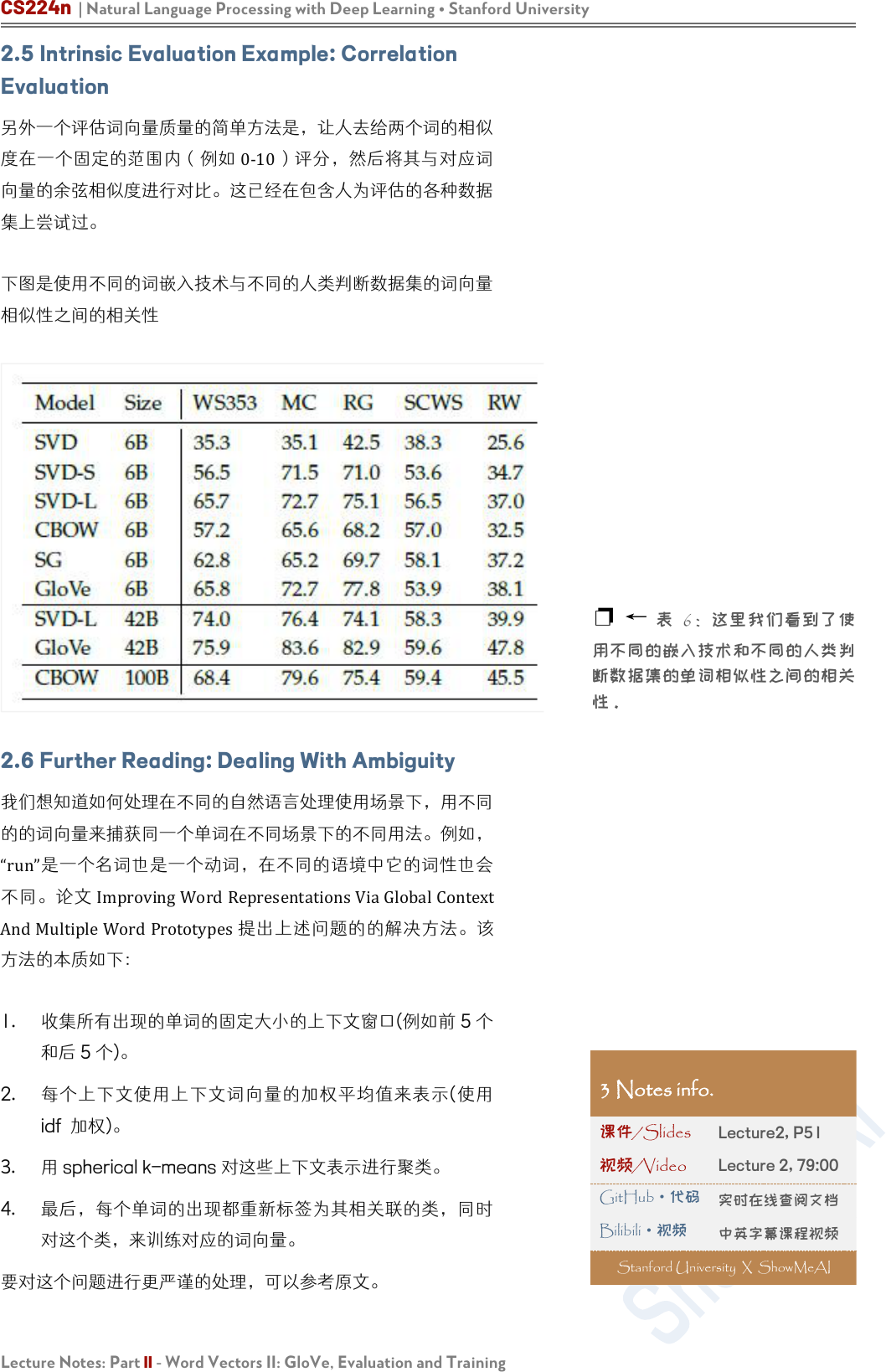CS224n | Natural Language Processing with Deep Learning Stanford University
Lecture Notes: Part II - Word Vectors II: GloVe, Evaluation and Training
2.5 Intrinsic Evaluation Example: Correlation
Evaluation

0-10

2.6 Further Reading: Dealing With Ambiguity

“run”
ImprovingWordRepresentationsViaGlobalContext
AndMultipleWordPrototypes

1. 收集所有现的单词固定大小上下文(例如 5

2. 使(使
idf 加权)
3. spherical k-means 对这些上下文表示进行聚类
4.

6使

3 Notes info.

Lecture2, P51

Lecture 2, 79:00
GitHub
·代码

Bilibili·视频

Stanford University X ShowMeAI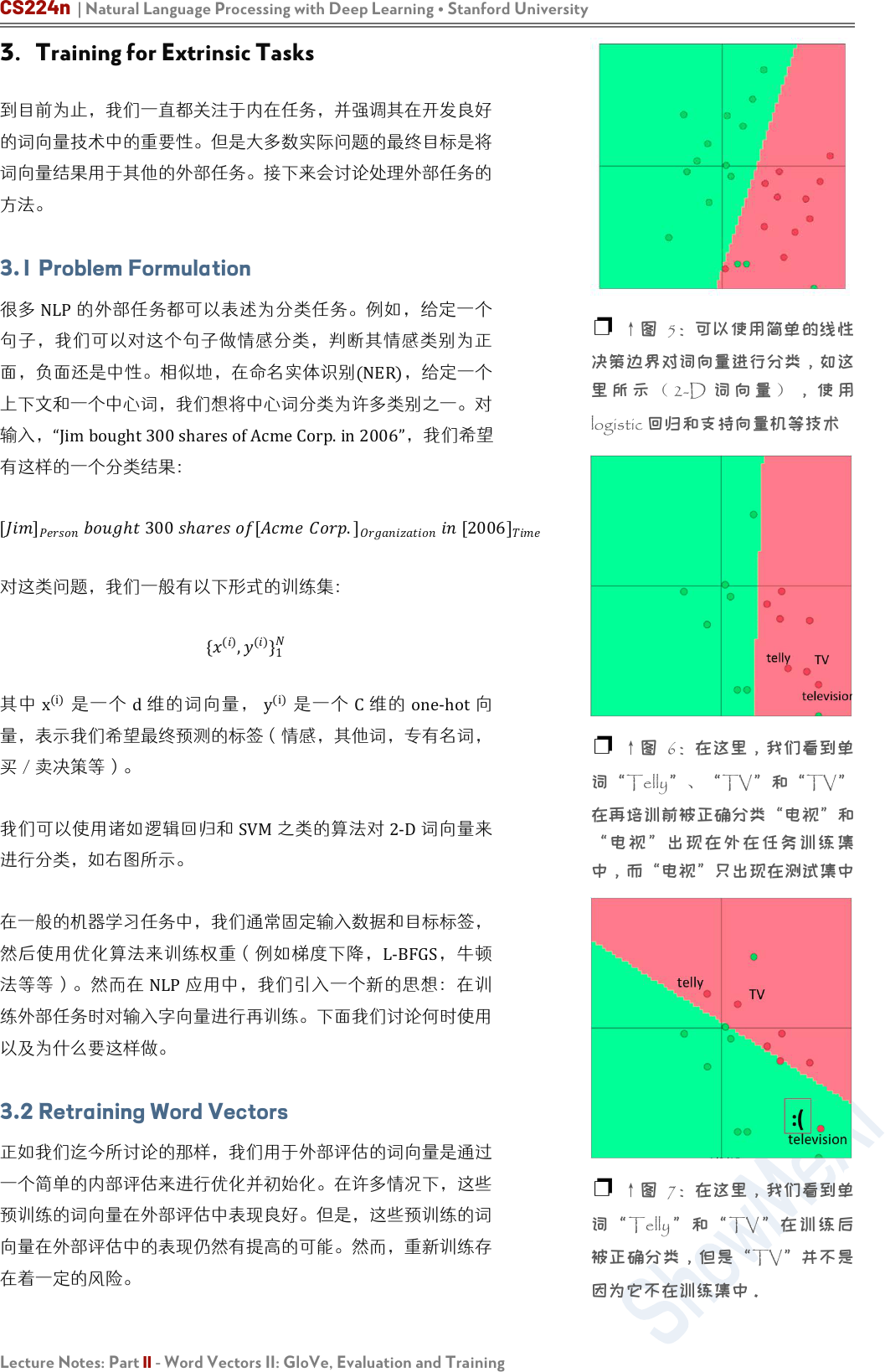CS224n | Natural Language Processing with Deep Learning Stanford University
Lecture Notes: Part II - Word Vectors II: GloVe, Evaluation and Training

3.1 Problem Formulation
NLP

[]

ℎ
300
ℎ
[
.]





{
()
,
()
}
1
x
(i)
d
y
(i)
C one-hot

NLP

3.2 Retraining Word Vectors

5可以使用单的线

2-D 使
logistic 回归和支持向量机等技术
6在这里，们看

7在这里，们看
TellyTV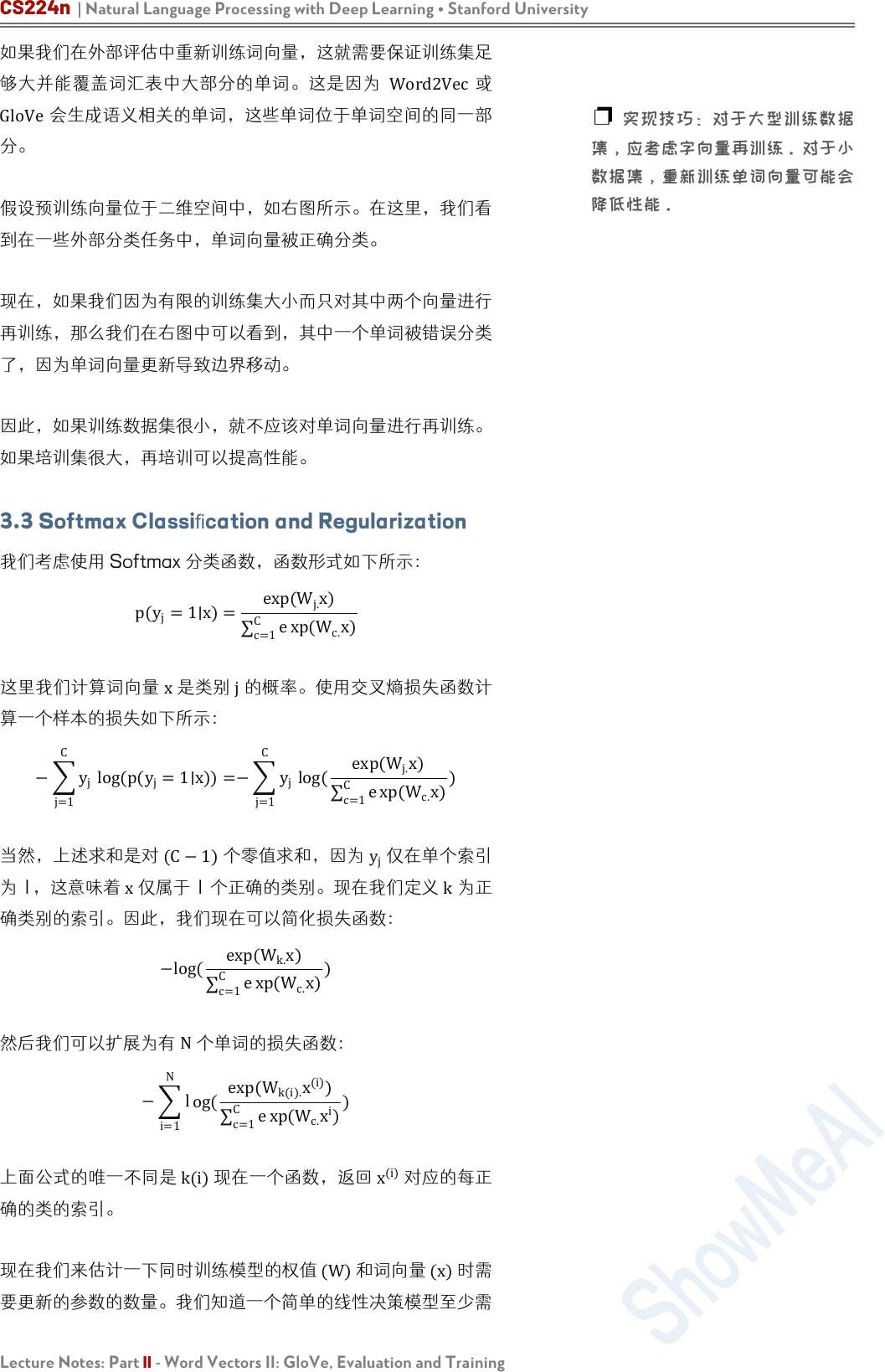CS224n | Natural Language Processing with Deep Learning Stanford University
Lecture Notes: Part II - Word Vectors II: GloVe, Evaluation and Training

Word2Vec
GloVe会生成语义相关的单词，这些单词位于单词空间的同一部

3.3 Softmax Classication and Regularization

p(y
j
=1∣x)=
exp(W
j.
x)
c=1
C
e
xp(W
c.
x)

j=1
C
y
j
log(p(y
j
=1∣x))=
j=1
C
y
j
log(
exp(W
j.
x)
c=1
C
e
xp(W
c.
x)
)

j

1，这意味 x仅属于 1 个正确的类别。现在我们定义
k

−log(
exp(W
k.
x)
c=1
C
e
xp(W
c.
x)
)

N

i=1
N
l
og(
exp(W
k(i).
x
(i)
)
c=1
C
e
xp(W
c.
x
i
)
)

(i)

(W)

(x)CS224n | Natural Language Processing with Deep Learning Stanford University
Lecture Notes: Part II - Word Vectors II: GloVe, Evaluation and Training

d

C

Cd

V

|V|

d

Cd+|V|
θ
J θ =
W.1
W.d
aardvark
zebra

=1
(
(
().
()
)
=1
(
.
)
)+
=1
⋅+||⋅
2

λ

3.4 Window Classification

“to sanction” “to
sanction”“topermit“topunish

(i)

window
(i)
8图是们有一个
2
Paris

,
,CS224n | Natural Language Processing with Deep Learning Stanford University
Lecture Notes: Part II - Word Vectors II: GloVe, Evaluation and Training

()
=
(−2)
(−1)
()
(+1)
(+2)


()
=
(−2)
(−1)
()
(+1)
(+2)

3.5 Non-linear Classifiers

GloVe Global Vectors for Word Representation

Improving Distributional Similarity with Lessons
Learned from Word Embeddings

Abstract

9在这里，们看

10CS224n | Natural Language Processing with Deep Learning Stanford University
Lecture Notes: Part II - Word Vectors II: GloVe, Evaluation and Training
Introduction
bag-of-contexts

Practical Recommendations

，我们发现它们在我们的设置中工作得很好：

SGNS 是健线每个务的

SGNS 适合更多的负样本

+c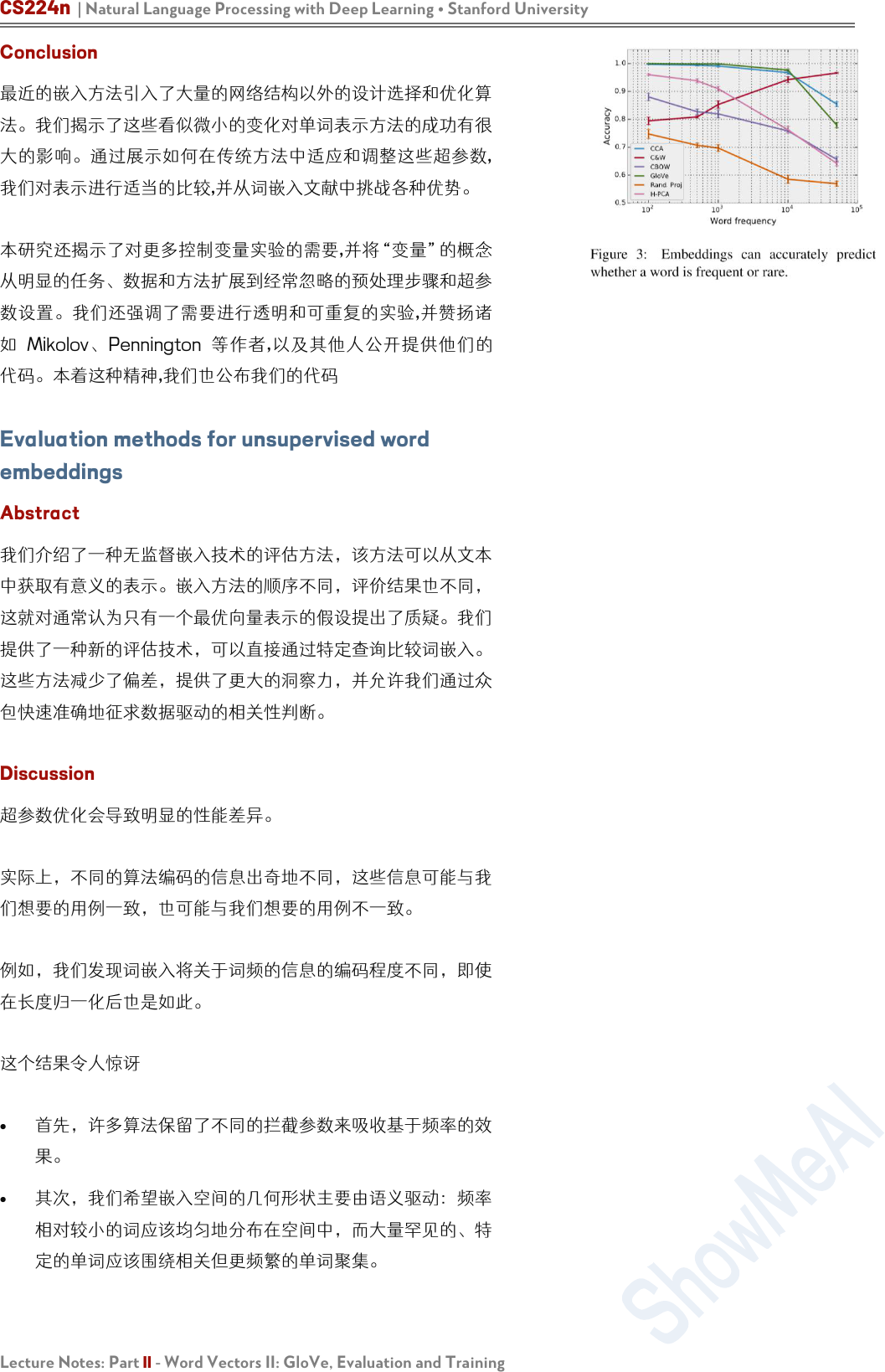CS224n | Natural Language Processing with Deep Learning Stanford University
Lecture Notes: Part II - Word Vectors II: GloVe, Evaluation and Training
Conclusion

,

,
MikolovPennington ,

Evaluation methods for unsupervised word
embeddings
Abstract

DiscussionCS224n | Natural Language Processing with Deep Learning Stanford University
Lecture Notes: Part II - Word Vectors II: GloVe, Evaluation and Training

50000 不等值频采样

(Turian et al., 2010)

,
GloVe TSCCA 在接 1000 的词 100%

GloVe CBOW
,

WordSim-
353 k = 1000

C&W

(nn-rank 1000 ·
corpus-rank
0.17
)

Related Work
Mikolov et al. (2013b)CS224n | Natural Language Processing with Deep Learning Stanford University
Lecture Notes: Part II - Word Vectors II: GloVe, Evaluation and Training

Faruqui and Dyer (2014)
Gaoetal.(2014)
Tsvetkov
(2015)提出了一种新的内在度量方法，该方法可以

(Collobert etal., 2011)
(Turianetal.2010)

(Chang et al. 2009)

(Newman et al. 2010)
ConclusionCS224n | Natural Language Processing with Deep Learning Stanford University
Lecture Notes: Part II - Word Vectors II: GloVe, Evaluation and Training

Machine Learning
Deep Learning
Natural Language Processing
Computer Vision
Knowledge Graphs
Stanford · CS229
Stanford · CS230
Stanford · CS224n
Stanford · CS231n
Stanford · CS520
# 系列内容 Awesome AI Courses Notes Cheatsheets

Machine Learning with Graphs
Deep Reinforcement Learning
Deep Learning for Self-Driving Cars
Stanford · CS224W
UCBerkeley · CS285
MIT · 6.S094
...
...
...
ShowMeAI 的分盖最名度 TOP20+AI 旨在习者整套